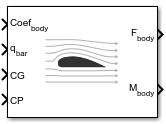# Aerodynamic Forces and Moments

Compute aerodynamic forces and moments using aerodynamic coefficients, dynamic pressure, center of gravity, center of pressure, and velocity

• Library:
• Aerospace Blockset / Aerodynamics

•## Description

The Aerodynamic Forces and Moments block computes the aerodynamic forces and moments about the center of gravity.

The Aerodynamic Forces and Moments block port labels change based on the coordinate system selected from the Input axes, Force axes, and Moment axes list.

## Limitations

• The default state of the block hides the Vb input port and assumes that the transformation is body-body.

• The center of gravity and the center of pressure are assumed to be in body axes.

• While this block has the ability to output forces and/or moments in the stability axes, the blocks in the Equations of Motion library are currently designed to accept forces and moments in either the body or wind axes only.

## Ports

### Input

expand all

Aerodynamic coefficients (in the chosen input axes) for forces and moments, specified as a vector. These coefficients are ordered into a vector depending on the choice of axes:

Input AxesInput Vector
Body(axial force Cx, side force Cy, normal force Cz, rolling moment Cl, pitching moment Cm, yawing moment Cn)
Stability(drag force CD(β=0), side force Cy, lift force CL, rolling moment Cl, pitching moment Cm, yawing moment Cn)
Wind(drag force CD, cross-wind force Cc, lift force CL, rolling moment Cl, pitching moment Cm, yawing moment Cn)

Data Types: `double`

Dynamic pressure, specified as a 1-by-3 array.

Data Types: `double`

Center of gravity, specified as a 1-by-3 vector.

Data Types: `double`

Center of pressure, specified as a 1-by-3 vector. This can also be taken as any general moment reference point as long as the rest of the model reflects the use of the moment reference point.

Data Types: `double`

Velocity in the body axes. specified as a 1-by-3 vector.

#### Dependencies

This port is enabled if the Input axes parameter is set to `Wind` or `Stability`.

Data Types: `double`

### Output

expand all

Aerodynamic forces (in the chosen output axes), returned as three-element vector, at the center of gravity in x-, y-, and z-axes.

Data Types: `double`

Aerodynamic moments (in the chosen output axes), returned as three-element vector, at the center of gravity in x-, y-, and z-axes.

Data Types: `double`

## Parameters

expand all

Coordinate system for input coefficients, specified as `Body` (default), `Stability`, or `Wind`.

#### Dependencies

Selecting `Stability` or `Wind` enables input port Port_5.

#### Programmatic Use

 Block Parameter: `inputAxes` Type: character vector Values: `'Body'` | `'Stability'` | `'Wind'` Default: `'Body'`

Coordinate system for aerodynamic force, specified as `Body` (default), `Stability`, or `Wind`.

#### Dependencies

Selecting `Stability` or `Wind` enables input port Port_5.

#### Programmatic Use

 Block Parameter: `outputForcesAxes` Type: character vector Values: `'Body'` | `'Stability'` | `'Wind'` Default: `'Body'`

Coordinate system for aerodynamic moment, specified as `Body` (default), `Stability`, or `Wind`.

#### Dependencies

Selecting `Stability` or `Wind` enables input port Port_5.

#### Programmatic Use

 Block Parameter: `outputMomentAxes` Type: character vector Values: `'Body'` | `'Stability'` | `'Wind'` Default: `'Body'`

Reference area for calculating aerodynamic forces and moments, specified as any double value.

#### Programmatic Use

 Block Parameter: `S` Type: character vector Values: any double value Default: `'1'`

Reference span for calculating aerodynamic moments in x-axes and z-axes, specified as any double value.

#### Programmatic Use

 Block Parameter: `b` Type: character vector Values: any double value Default: `'1'`

Reference length for calculating aerodynamic moment in the y-axes, specified as any double value.

#### Programmatic Use

 Block Parameter: `cbar` Type: character vector Values: any double value Default: `'1'`

## Algorithms

Let α be the angle of attack and β the sideslip. The rotation from body to stability axes:

`${C}_{s←b}=\left[\begin{array}{ccc}\mathrm{cos}\left(\alpha \right)& 0& \mathrm{sin}\left(\alpha \right)\\ 0& 1& 0\\ -\mathrm{sin}\left(\alpha \right)& 0& \mathrm{cos}\left(\alpha \right)\end{array}\right]$`

can be combined with the rotation from stability to wind axes:

`${C}_{w←s}=\left[\begin{array}{ccc}\mathrm{cos}\left(\beta \right)& \mathrm{sin}\left(\beta \right)& 0\\ -\mathrm{sin}\left(\beta \right)& \mathrm{cos}\left(\beta \right)& 0\\ 0& 0& 1\end{array}\right]$`

to yield the net rotation from body to wind axes:

`${C}_{w←b}=\left[\begin{array}{ccc}\mathrm{cos}\left(\alpha \right)\mathrm{cos}\left(\beta \right)& \mathrm{sin}\left(\beta \right)& \mathrm{sin}\left(\alpha \right)\mathrm{cos}\left(\beta \right)\\ -\mathrm{cos}\left(\alpha \right)\mathrm{sin}\left(\beta \right)& \mathrm{cos}\left(\beta \right)& -\mathrm{sin}\left(\alpha \right)\mathrm{sin}\left(\beta \right)\\ -\mathrm{sin}\left(\alpha \right)& 0& \mathrm{cos}\left(\alpha \right)\end{array}\right]$`

Moment coefficients have the same notation in all systems. Force coefficients are given below. Note there are no specific symbols for stability-axes force components. However, the stability axes have two components that are unchanged from the other axes.

`${F}_{A}^{w}\equiv \left[\begin{array}{c}-D\\ -C\\ -L\end{array}\right]={C}_{w←b}\cdot \left[\begin{array}{c}{X}_{A}\\ {Y}_{A}\\ {Z}_{A}\end{array}\right]\equiv {C}_{w←b}\cdot {F}_{A}^{b}$`
Components/Axesxyz
WindCDCCCL
StabilityCYCL
BodyCXCYCZ (–CN)

Given these definitions, to account for the standard definitions of D, C, Y (where Y = -C), and L, force coefficients in the wind axes are multiplied by the negative identity diag(-1, -1, -1). Forces coefficients in the stability axes are multiplied by diag(-1, 1, -1). CN and CX are, respectively, the normal and axial force coefficients (CN = -CZ).

 Stevens, B. L., and F. L. Lewis, Aircraft Control and Simulation, John Wiley & Sons, New York, 1992

## SupportGet trial now